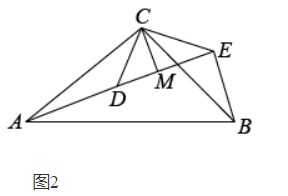(1)问题发现:(2)解决问题: 如图2，若 $\triangle A C B$ 和 $\triangle D C E$ 均为等腰直角三角形， $\angle A C B=\angle D C E=90^{\circ}$ ，点 $A ， D ， E$ 在同一条直线上，CM为 $\triangle D C E$ 中 $D E$ 边上的高，连接 $B E$ ，请判断 $\angle A E B$ 的度数及线段 $C M ， A E ， B E$ 之间的数量关系并说明理由.【答案】 （1）证明: $\because \triangle A B C$ 和 $\triangle A D E$ 是顶角相等的等腰三角形，
\begin{aligned} & \therefore A B=A C, A D=A E, \angle B A C=\angle D A E ， \\ & \therefore \angle B A C-\angle C A D=\angle D A E-\angle C A D ， \\ & \therefore \angle B A D=\angle C A E . \end{aligned}

\begin{aligned} & \left\{\begin{array}{l} A B=A C \\ \angle B A D=\angle C A E \\ A D=A E \end{array}\right. \\ & \therefore \triangle B A D \cong \triangle C A E(S A S) \\ & \therefore B D=C E \end{aligned}

(2) 解: $\angle A E B=90^{\circ} ， A E=B E+2 C M$,

\begin{aligned} & \therefore A D=B E ， \angle A D C=\angle B E C, \\ & \because \triangle C D E \text { 是等䁏直角三角形， } \\ & \therefore \angle C D E=\angle C E D=45^{\circ}, \\ & \therefore \angle A D C=180^{\circ}-\angle C D E=135^{\circ}, \\ & \therefore \angle B E C=\angle A D C=135^{\circ}, \\ & \therefore \angle A E B=\angle B E C-\angle C E D=135^{\circ}-45^{\circ}=90^{\circ} . \\ & \because C D=C E ， C M \perp D E ， \\ & \therefore D M=M E . \\ & \because \angle D C E=90^{\circ} ， \\ & \therefore D M=M E=C M ， \\ & \therefore D E=2 C M . \\ & \therefore A E=A D+D E=B E+2 C M . \end{aligned}